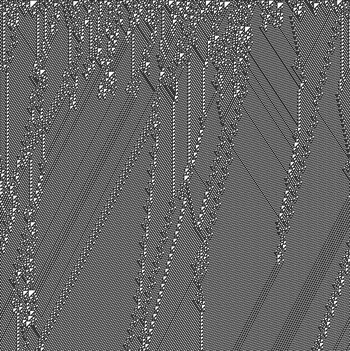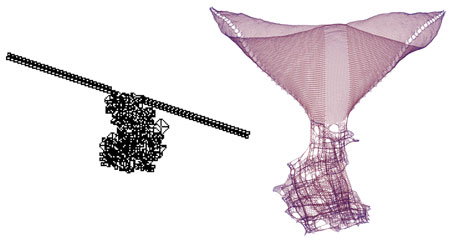# Buiding Discrete Spacetimes by Simple Deterministic Computations

by Tommaso Bolognesi

The Computational Universe conjecture relates complexity in physics with emergence in computation. Our current research efforts are meant to put the surprisingly powerful notion of (computational) emergence at the service of recent quantum gravity theories, with special attention to the Causal Set Programme, which assumes causality among events as the most fundamental structure of spacetime, and causal sets as the most appropriate way to describe it.

Our physical universe is discrete, finite, unbounded, deterministic and computational. Of course most readers will disagree with most of these attributions, but a number of researchers in the last few decades have been willing to take at least some of them as stimulating ‘working hypotheses’ for exploring alternative physical theories whose basic ideas could hardly be beaten in terms of simplicity.

Discrete means that there exists a tiniest scale at which the fabric of space (and also of space-time) appears as a pomegranate, made of indivisible atoms, or seeds; this viewpoint is adopted in theories such Loop Quantum Gravity and in the so called Causal Sets Programme, and is reflected in models such as Penrose’s spin networks and spin foams.

Finite means that the number of seeds in the pomegranate is finite, say 10234 (as of year 2010 a.c.)

Unbounded means that new seeds keep popping up as the universe evolves, but always in finite quantities, perhaps one at a time.

Deterministic means that at this ultimate level, reality obeys precise rules that do not involve any coin flipping; we are thus assuming that God indeed does not play dice, and we look with great hope at some recent efforts, eg by G. ‘tHooft, that try to unveil a deterministic layer under the apparent probabilistic nature of Quantum Mechanics.

Computational means that these rules can be implemented and executed step by step on a digital computer; this does not mean that we have to postulate the existence of a divine digital Computer that sits in some outer space and runs the program for our universe, for the same reason that under a continuous mathematics viewpoint we do not need to postulate the existence of a divine analog Computer that runs the Navier-Stokes differential equations of fluid dynamics.

Eminent physicist Richard Feynman is one of the scientists that have been intrigued by the idea of a discrete, computational universe. The following is a famous passage from his 1964 Cornell Lectures (‘The Character of Physical Law’):’It always bothers me that, according to the laws as we understand them today, it takes a computing machine an infinite number of logical operations to figure out what goes on in no matter how tiny a region of space, and no matter how tiny a region of time. How can all that be going on in that tiny space? Why should it take an infinite amount of logic to figure out what one tiny piece of spacetime is going to do? So I have often made the hypothesis that ultimately physics will not require a mathematical statement, that in the end the machinery will be revealed, and the laws will turn out to be simple, like the chequer board with all its apparent complexities.’

What type of complexity can appear, or emerge, by playing a simple game on a checkerboard? An answer is provided by Conway’s Game of Life, a well known example of two-dimensional cellular automaton that became popular in the 1970’s. By letting all elements of a square array of cells obey, synchronously, the same simple rule, which only refers to the color (black or white) of the cell and of its eight neighbors, one obtains surprisingly complex populations of moving patterns (‘gliders’, kites’, ‘darts’, …) that suggest a lively aerial scenario.Figure 1: The artificial ‘particles’ emergent from the computations of Elementary Cellular Automaton n. 110 are reminiscent of ‘real’ scattering phenomena in physics, and at the same time achieve computational universality (Turing-completeness

The fact that simple deterministic computational rules can originate highly complex patterns and dynamics has been further explored and popularized by Stephen Wolfram, who showed that even simpler models of computation, notably one-dimensional, two-color cellular automata (ECA), can exhibit very complex behaviours. Two surprising examples are provided by ECA n. 30, with its pseudo-random computations, and ECA n. 110, with its emergent particles. The latter is illustrated in Figure 1, where the configurations of the 1-D array of cells are stacked, so that the horizontal and vertical dimensions correspond, respectively, to space and time. While these particles and their behaviors are clearly reminiscent of scattering in ‘real’ physical phenomena, Cook and Wolfram were able to formally prove that they can also simulate any Turing machine (like Conway’s Game of Life), thus turning the device into a universal computer.

There are several ways in which one can represent the computations of a given model, devise complexity indicators characterizing their behaviours, and detect the emergence of interesting features such as pseudorandomness or interacting particles. A particularly attractive way to do this is to consider the ‘causal set’ associated with the computation, which is formed by a set of events and a set of causal relations among them. This approach appears as particularly appropriate for applications in fundamental physics, since causal sets are regarded as one of the most appropriate models of discrete, physical space-time.

Obtaining causal sets from the computations of simple models such as Turing machines, network mobile automata, or graph rewrite systems, is fairly easy.

In doing so we may obtain two attractive results. On one hand, these ‘algorithmic’ causal sets might represent, under the ‘Computational Universe perspective, the only information of physical relevance that we can extract from the considered models of computation, and a way to abstract from the internal machinery of the latter. On the other hand, this approach might represent a fully deterministic, radical alternative to the probabilistic techniques currently adopted in the Causal Set Programme for growing discrete spacetimes.

If pseudo-particles and pseudo-randomness emerge from simple deterministic computations, we may expect to spot similar phenomena also in the causal sets derived from them. An example of two coupled particles that we have discovered, emerging from the computation of a 2D Turing machine (one moving on a checkerboard), is shown in Figure 2.Figure 2: Emergence of two coupled particles in the final configuration of a 2D Turing machine computation (left) and in the corresponding causal set (right).